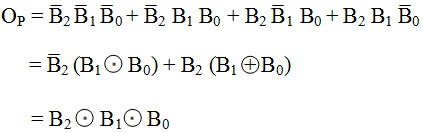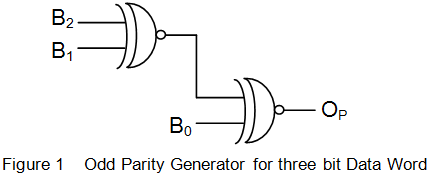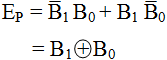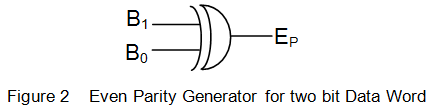# Parity Generator

Current world is undoubtedly an electronic world full of different types of systems performing various kinds of functions. These systems need to communicate amongst themselves so as to achieve their intended objectives. The actions undertaken by them heavily relay on the data received/transmitted by/from them. Say for example, suppose we are landing a plane and send 0010000 to the system to drag the wheels out.
Now, imagine that our bit pattern gets corrupted along the channel and is received by the system as 0010100. This might cause the system to either discard its input (if its invalid) or perform altogether a different action from the intended one. In either case, the result will be the disaster, just as our one bit got altered. This (rather exaggerated) example indicates the importance of error-free data communication. One way of achieving this is the use of parity generators and checkers.

Parity checking is a method in which an extra bit called parity bit is appended, usually at the MSB, of the data stream which needs to be transmitted. Now, how to decide whether to add 1 or 0? This depends on whether we desire to have odd parity or even parity.

### Odd Parity

This is the case wherein the number of ones in the bit stream sent (data bits in conjunction with parity-bit) has to be maintained as an odd number. That is, suppose we have our bit stream as 1001011, then the parity would be generated as 1 such that the number of ones in the resulting bit-stream (= 11001011) is 5, an odd number. Reasoning in similar fashion, we can say that for the data sequence of 1001010, the parity-bit should necessarily be 0.

### Even Parity

In case if we opt for even parity, then we need to ensure that the total number of ones in the bit stream, including the parity bit, becomes an even number. For example, if the data string is 1001011, then the parity-bit would be 0; while if it is 1001010, then parity-bit should be 1.
Now, let us try to design a digital circuit which can help us generate our parity-bit.

## Odd Parity Generator

Let us assume that we have a three-bit data sequence (B2B1B0) which needs to be communicated. Table I shows the parity bits (OP) which must be generated by our circuit for each combination of input bits.
Table I

 B2 B1 B0 OP 0 0 0 1 0 0 1 0 0 1 0 0 0 1 1 1 1 0 0 0 1 0 1 1 1 1 0 1 1 1 1 0

From this, we can find the expression for P in terms of B2, B1 and B0 asThis means that we can build an odd parity generator for three bits of data word using two XNOR gates. The design realized is as shown by Figure 1.## Even Parity Generator

Let us now design an even parity (EP) generator for two-bit data word B1B0. The associated truth table is as shown by Table II.
Table II

 B1 B0 EP 0 0 0 0 1 1 1 0 1 1 1 0

Thus we haveThis leads to the digital circuit as shown in Figure 2.This kind of parity generation is employed in SPI buses and UART transmissions.

Want To Learn Faster? 🎓
Get electrical articles delivered to your inbox every week.
No credit card required—it’s 100% free.